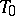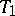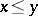# Kolmogorov axiom

(diff) ← Older revision | Latest revision (diff) | Newer revision → (diff)-axiom
The weakest of all separation axioms (cf. Separation axiom) in general topology; introduced by A.N. Kolmogorov. A topological space satisfies this axiom, i.e. it is a-space or a Kolmogorov space, if for any two distinct points of the space there exists an open set containing one of the points but not containing the other. If it is required that each of the two (arbitrarily given) points be contained in an open set not containing the other, then one obtains the next stronger separation axiom, called the-axiom; topological spaces satisfying it are called-spaces. The simplest example of a-space that is not a-space is the connected digon.
In a-space, a singleton need not be closed;-spaces can be defined as-spaces for which all singletons are closed. A-space in which the intersection of any number of open sets is open is called a discrete space (in the broad sense). It is precisely in these spaces that the closure of the union of any family of sets is the same as the union of the closures of these sets. In any discrete space and even in any Kolmogorov space one can define a (partial) order between its pointsand:ifis contained in the closure of the singleton consisting of the point. Conversely, if one defines in an arbitrary partially ordered set the closure of any pointas the set of all pointsand takes for the closure of a set the union of the closures of all of its points, then one obtains a discrete space. Thus, the study of discrete spaces is equivalent to that of partially ordered sets. Simplicial (and more general) complexes in combinatorial topology are important examples of discrete spaces: For two simplices,the order relationmeans thatis a face (possibly improper) of the simplex.